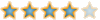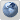The Laminar Project Forum
Would you like to react to this message? Create an account in a few clicks or log in to continue.

# How to size your pump##How to size your pump

There have been several questions on how to size your pump. Easy! BIG! If that isn't descriptive enough for you, I've put together some equations and an example so that you can calculate it on your own.
There are probably a number of different ways to figure out the size of your pump this is just one way to do it.

There are a few things that must be known in order to figure it out. These are called givens.

GIVENS:

Nozzle output (dnozzle): The output size of your nozzle, the water diameter.
Desire Height (H) and Distance (D) of the arc: How far do you want it to throw the water.
Gravity g: 32.2 ft/s^2 or 9.81 m/s^2

Equations:

vy = sqrt(H*g/4) Eq 1

vx = D*g/(2*vy) Eq 2

v = sqrt(vy^2 + vx^2) Eq 3

Q=V*pi*(dnozzle/2)^2 Eq 4

Note: These are just the general equations. When using these equations you must make sure that your units are the same (i.e. don't mix inch with feet etc).

Example (English Units)

Desired 6' (H) by 12' (D) arc. With a 1/2" output (.041667 ft). It's probably best to convert everything to feet. So the

vy = sqrt(H*g/4) = sqrt(6*32.2/4) = 6.94 ft/s Eq 1

vx = D*g/(2*vy) = 12*32.2/(2*6.94) = 27.83 ft/s Eq 2

v = sqrt(vy^2 + vx^2) = sqrt(6.94^2 + 27.83^2) = 28.68 ft/s Eq 3

Q=v*pi*(dnozzle/2)^2 = 28.68*pi*(.041667/2)^2 = .039 ft^3/s Eq 4

Pumps usually are in gallons/hour so we need to convert the answer from Eq 4. This can be done by multiplying

.039 ft^3 * 7.48051948 gallons/ft^3 = .2925 gallons/s

.2925 gallons/s * 60 seconds/minute * 60 mintues/hour = 1053 gallons/hour

Note: This does not include any losses due to the constriction of the tubing/piping, angles, the nozzle, the filters etc. It will give you a ball park figure. If you need the exact arc oversize the pump and put in a throttling valve (or ball valve) to get the exact distance.

I'll update this later for SI units.John
Nozzle MasterPosts : 450
Join date : 2009-04-03
Age : 44
Location : UtahPermissions in this forum:
You cannot reply to topics in this forum